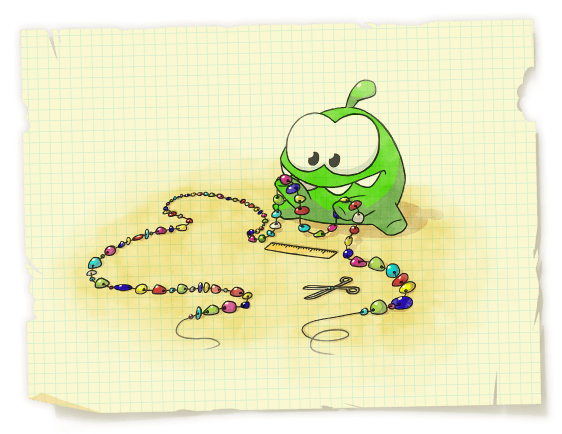# Om Nom and Necklace

One day Om Nom found a thread with n beads of different colors. He decided to cut the first several beads from this thread to make a bead necklace and present it to his girlfriend Om Nelly.Om Nom knows that his girlfriend loves beautiful patterns. That’s why he wants the beads on the necklace to form a regular pattern. A sequence of beads S is regular if it can be represented as S = A + B + A + B + A + … + A + B + A, where A and B are some bead sequences, “ + ” is the concatenation of sequences, there are exactly 2k + 1 summands in this sum, among which there are k + 1 ” A” summands and k ” B” summands that follow in alternating order. Om Nelly knows that her friend is an eager mathematician, so she doesn’t mind if A or B is an empty sequence.

Help Om Nom determine in which ways he can cut off the first several beads from the found thread (at least one; probably, all) so that they form a regular pattern. When Om Nom cuts off the beads, he doesn’t change their order.

Input

The first line contains two integers nk (1 ≤ n, k ≤ 1 000 000) — the number of beads on the thread that Om Nom found and number k from the definition of the regular sequence above.

The second line contains the sequence of n lowercase Latin letters that represent the colors of the beads. Each color corresponds to a single letter.

Output

Print a string consisting of n zeroes and ones. Position i (1 ≤ i ≤ n) must contain either number one if the first i beads on the thread form a regular sequence, or a zero otherwise.

Examples

input

7 2bcabcab

output

0000011

input

21 2ababaababaababaababaa

output

000110000111111000011

Note

In the first sample test a regular sequence is both a sequence of the first 6 beads (we can take A = ””, B = ”bca”), and a sequence of the first 7 beads (we can take A = ”b”, B = ”ca”).

In the second sample test, for example, a sequence of the first 13 beads is regular, if we take A = ”aba”, B = ”ba”.

Solution:

#include <bits/stdc++.h>

using namespace std;

const int N = 1000010;
const int LOG = 22;

char s[N];
int p[N];
int pr[N][LOG];

int main() {
int n, k;
scanf("%d %d", &n, &k);
scanf("%s", s + 1);
int kk = 0;
p = 0;
for (int i = 2; i <= n; i++) {
while (kk > 0 && s[i] != s[kk + 1]) kk = p[kk];
if (s[i] == s[kk + 1]) kk++;
p[i] = kk;
}
p = 0;
for (int i = 0; i <= n; i++) {
pr[i] = p[i];
}
for (int j = 1; j < LOG; j++) {
for (int i = 0; i <= n; i++) {
pr[i][j] = pr[pr[i][j - 1]][j - 1];
}
}
for (int len = 1; len <= n; len++) {
int from = (((long long)(k - 1)) * len + k - 1) / k;
int to = (((long long)k) * len) / (k + 1);
int t = len;
for (int j = LOG - 1; j >= 0; j--) {
if (pr[t][j] > to) {
t = pr[t][j];
}
}
t = pr[t];
if (from <= t && t <= to) {
putchar('1');
} else {
putchar('0');
}
}
return 0;
}